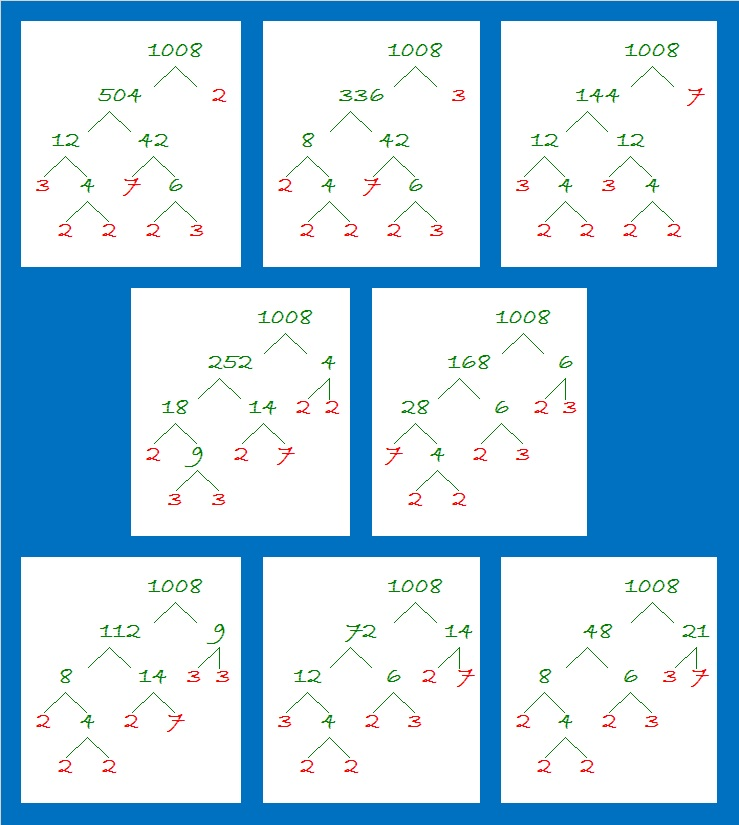# A Forest of 1008 Factor Trees

The number 1008 has so many factors that I just had to make a forest of some of its MANY possible factor trees. 1008 has fifteen factor pairs. I’ve made a factor tree for every factor pair, except 1 × 1008. I’ll start off with these short, wide, beautiful trees that feature six of 1008’s factor pairs:Notice that no matter what factor pair we use, each tree has the same prime factors that I have highlighted in red. Even this lean, thin very-easy-to-read tree uses those same prime factors:Finally, here are eight more factor trees that begin with the other eight factor pairs for 1008. They aren’t as good-looking as all the trees above, but they still work as factor trees and help us find those same red prime factors for 1008:Here are some other facts about the number 1008:

1008 is the sum of ten consecutive prime numbers:
79 + 83 + 89 + 97 + 101 + 103 + 107 + 109 + 113 + 127 = 1008

1008 looks interesting when written in some other bases:
It’s 33300 in BASE 4 because 3(4⁴) + 3(4³) + 3(4²) = 3(256 + 64 + 16) = 3(336) = 1008,
4400 in BASE 6 because 4(6³) + 4(6²) = 4(216 + 36) = 4(252) = 1008,
700 in BASE 12 because 7(12²) = 7(144) = 1008
SS in BASE 35 (S is 28 base 10) because 28(35) + 28(1) = 28(36) = 1008
S0 in BASE 36 because 28(36) = 1008

• 1008 is a composite number.
• Prime factorization: 1008 = 2 × 2 × 2 × 2 × 3 × 3 × 7, which can be written 1008 = 2⁴ × 3² × 7
• The exponents in the prime factorization are 4, 2 and 1. Adding one to each and multiplying we get (4 + 1)(2 + 1)(1 + 1) = 5 × 3 × 2 = 30. Therefore 1008 has exactly 30 factors.
• Factors of 1008: 1, 2, 3, 4, 6, 7, 8, 9, 12, 14, 16, 18, 21, 24, 28, 36, 42, 48, 56, 63, 72, 84, 112, 126, 144, 168, 252, 336, 504, 1008
• Factor pairs: 1008 = 1 × 1008, 2 × 504, 3 × 336, 4 × 252, 6 × 168, 7 × 144, 8 × 126, 9 × 112, 12 × 84, 14 × 72, 16 × 63, 18 × 56, 21 × 48, 24 × 42, or 28 × 36
• Taking the factor pair with the largest square number factor, we get √1008 = (√144)(√7) = 12√7 ≈ 31.74902This site uses Akismet to reduce spam. Learn how your comment data is processed.